## Vector Addition and Subtraction: Graphical Methods

### Learning Objectives

By the end of this section, you will be able to:

• Understand the rules of vector addition, subtraction, and multiplication.
• Apply graphical methods of vector addition and subtraction to determine the displacement of moving objects.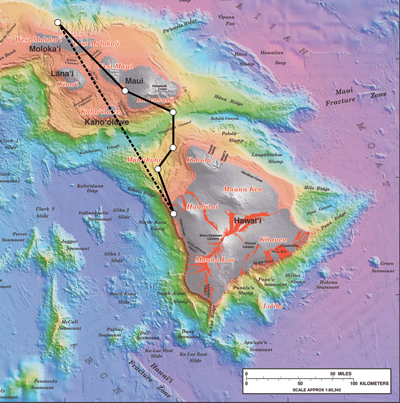Figure 1. Displacement can be determined graphically using a scale map, such as this one of the Hawaiian Islands. A journey from Hawai’i to Moloka’i has a number of legs, or journey segments. These segments can be added graphically with a ruler to determine the total two-dimensional displacement of the journey. (credit: US Geological Survey)

## Vectors in Two Dimensions

A vector is a quantity that has magnitude and direction. Displacement, velocity, acceleration, and force, for example, are all vectors. In one-dimensional, or straight-line, motion, the direction of a vector can be given simply by a plus or minus sign. In two dimensions (2-d), however, we specify the direction of a vector relative to some reference frame (i.e., coordinate system), using an arrow having length proportional to the vector’s magnitude and pointing in the direction of the vector.

Figure 2 shows such a graphical representation of a vector, using as an example the total displacement for the person walking in a city considered in Kinematics in Two Dimensions: An Introduction. We shall use the notation that a boldface symbol, such as D, stands for a vector. Its magnitude is represented by the symbol in italics, D, and its direction by θ.

### Vectors in this Text

In this text, we will represent a vector with a boldface variable. For example, we will represent the quantity force with the vector F, which has both magnitude and direction. The magnitude of the vector will be represented by a variable in italics, such as F, and the direction of the variable will be given by an angle θ.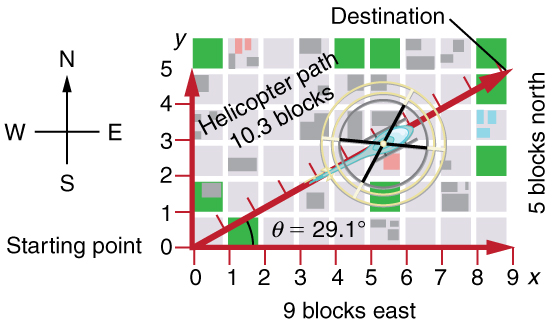Figure 2. A person walks 9 blocks east and 5 blocks north. The displacement is 10.3 blocks at an angle 29.1º north of east.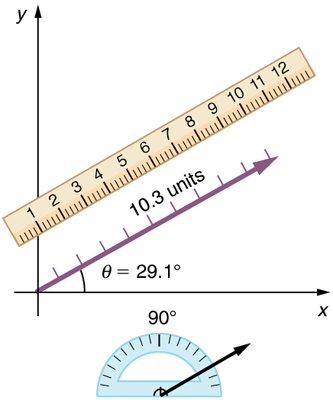Figure 3. To describe the resultant vector for the person walking in a city considered in Figure 2 graphically, draw an arrow to represent the total displacement vector D. Using a protractor, draw a line at an angle θ relative to the east-west axis. The length D of the arrow is proportional to the vector’s magnitude and is measured along the line with a ruler. In this example, the magnitude D of the vector is 10.3 units, and the direction θ is 29.1º north of east.

The head-to-tail method is a graphical way to add vectors, described in Figure 4 below and in the steps following. The tail of the vector is the starting point of the vector, and the head (or tip) of a vector is the final, pointed end of the arrow.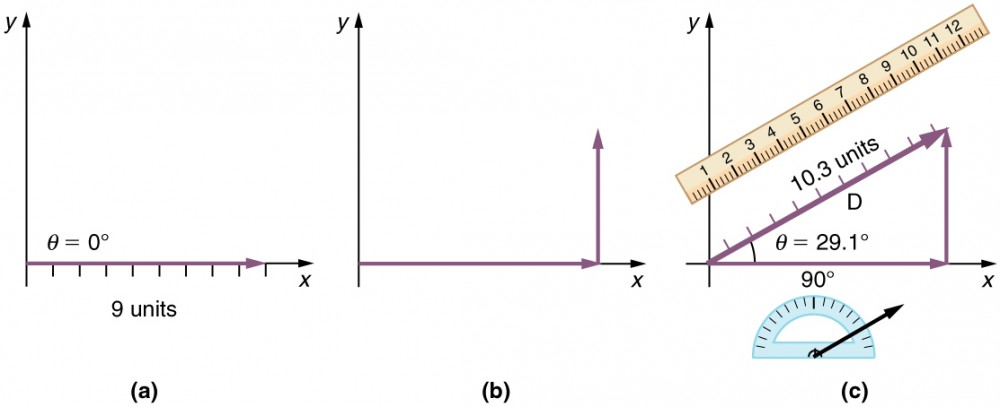Figure 4. Head-to-Tail Method: The head-to-tail method of graphically adding vectors is illustrated for the two displacements of the person walking in a city considered in Figure 2. (a) Draw a vector representing the displacement to the east. (b) Draw a vector representing the displacement to the north. The tail of this vector should originate from the head of the first, east-pointing vector. (c) Draw a line from the tail of the east-pointing vector to the head of the north-pointing vector to form the sum or resultant vector D. The length of the arrow D is proportional to the vector’s magnitude and is measured to be 10.3 units. Its direction, described as the angle with respect to the east (or horizontal axis) θ is measured with a protractor to be 29. 1º.

Step 1. Draw an arrow to represent the first vector (9 blocks to the east) using a ruler and protractor.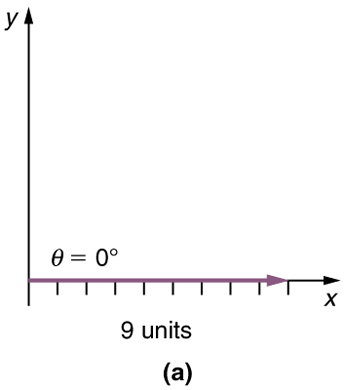Figure 5

Step 2. Now draw an arrow to represent the second vector (5 blocks to the north). Place the tail of the second vector at the head of the first vector.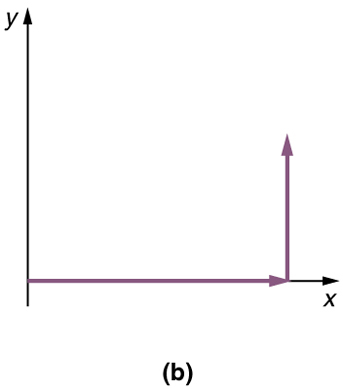Figure 6

Step 3. If there are more than two vectors, continue this process for each vector to be added. Note that in our example, we have only two vectors, so we have finished placing arrows tip to tail.

Step 4. Draw an arrow from the tail of the first vector to the head of the last vector. This is the resultant, or the sum, of the other vectors.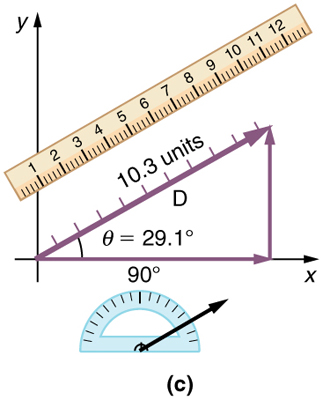Figure 7

Step 5. To get the magnitude of the resultant, measure its length with a ruler. (Note that in most calculations, we will use the Pythagorean theorem to determine this length.)

Step 6. To get the direction of the resultant, measure the angle it makes with the reference frame using a protractor. (Note that in most calculations, we will use trigonometric relationships to determine this angle.)

The graphical addition of vectors is limited in accuracy only by the precision with which the drawings can be made and the precision of the measuring tools. It is valid for any number of vectors.

### Example 1. Adding Vectors Graphically Using the Head-to-Tail Method: A Woman Takes a Walk

Use the graphical technique for adding vectors to find the total displacement of a person who walks the following three paths (displacements) on a flat field. First, she walks 25.0 m in a direction 49.0º north of east. Then, she walks 23.0 m heading 15.0º north of east. Finally, she turns and walks 32.0 m in a direction 68.0° south of east.

#### Strategy

Represent each displacement vector graphically with an arrow, labeling the first A, the second B, and the third C, making the lengths proportional to the distance and the directions as specified relative to an east-west line. The head-to-tail method outlined above will give a way to determine the magnitude and direction of the resultant displacement, denoted R.

#### Solution

(1) Draw the three displacement vectors.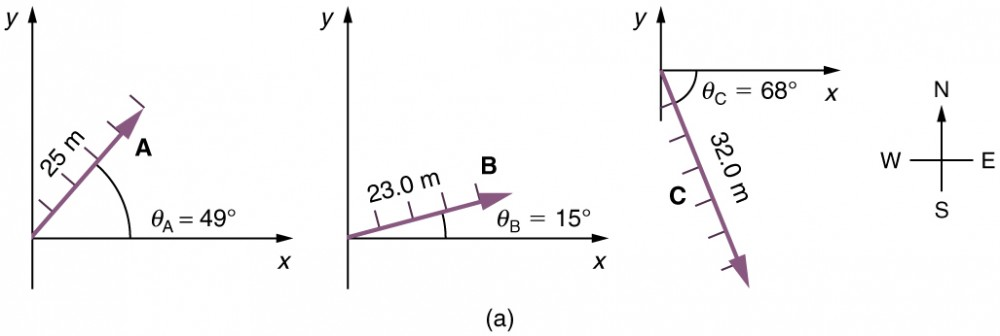Figure 8.

(2) Place the vectors head to tail retaining both their initial magnitude and direction.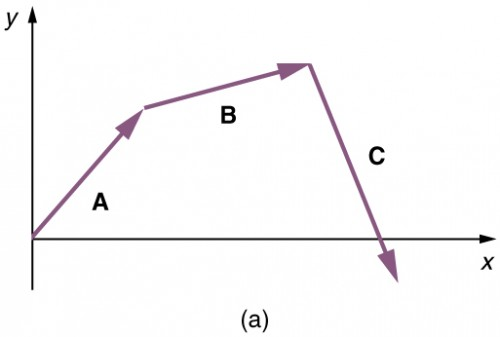Figure 9.

(3) Draw the resultant vector, R.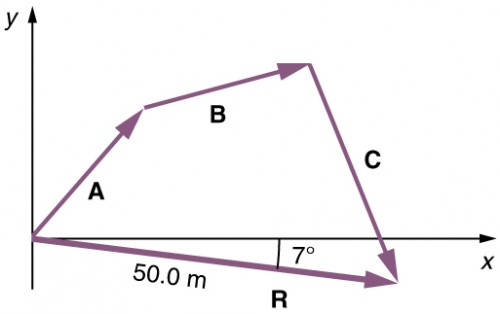Figure 10.

(4) Use a ruler to measure the magnitude of R, and a protractor to measure the direction of R. While the direction of the vector can be specified in many ways, the easiest way is to measure the angle between the vector and the nearest horizontal or vertical axis. Since the resultant vector is south of the eastward pointing axis, we flip the protractor upside down and measure the angle between the eastward axis and the vector.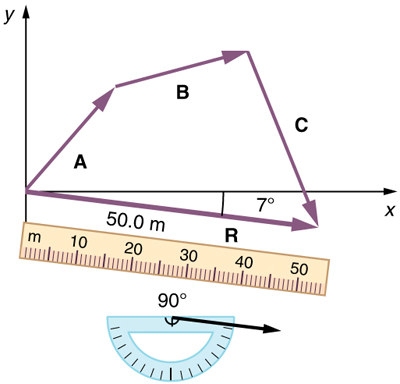Figure 11

In this case, the total displacement R is seen to have a magnitude of 50.0 m and to lie in a direction 7.0º south of east. By using its magnitude and direction, this vector can be expressed as R = 50.0 m and θ = 7.0º south of east.

#### Discussion

The head-to-tail graphical method of vector addition works for any number of vectors. It is also important to note that the resultant is independent of the order in which the vectors are added. Therefore, we could add the vectors in any order as illustrated in Figure 12 and we will still get the same solution.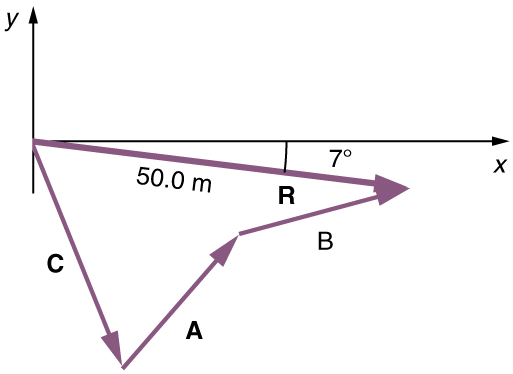Figure 12.

Here, we see that when the same vectors are added in a different order, the result is the same. This characteristic is true in every case and is an important characteristic of vectors. Vector addition is commutative. Vectors can be added in any order.

A + B = B + A.

(This is true for the addition of ordinary numbers as well—you get the same result whether you add 2 + 3 or 3 + 2, for example).

This video can be used for review.  It includes vector basics – drawing vectors/vector addition. You’ll learn about the basic notion of a vector, how to add vectors together graphically, as well as what it means graphically to multiply a vector by a scalar.

## Vector Subtraction

Vector subtraction is a straightforward extension of vector addition. To define subtraction (say we want to subtract B from A , written A – B , we must first define what we mean by subtraction. The negative of a vector B is defined to be –B; that is, graphically the negative of any vector has the same magnitude but the opposite direction, as shown in Figure 13. In other words, B has the same length as –B, but points in the opposite direction. Essentially, we just flip the vector so it points in the opposite direction.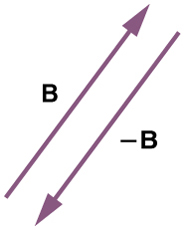Figure 13. The negative of a vector is just another vector of the same magnitude but pointing in the opposite direction. So B is the negative of –B; it has the same length but opposite direction.

The subtraction of vector B from vector A is then simply defined to be the addition of –B to A. Note that vector subtraction is the addition of a negative vector. The order of subtraction does not affect the results.

A – B = A + (-B)

This is analogous to the subtraction of scalars (where, for example, 5 – 2 = 5 + (–2)). Again, the result is independent of the order in which the subtraction is made. When vectors are subtracted graphically, the techniques outlined above are used, as the following example illustrates.

### Example 2. Subtracting Vectors Graphically: A Woman Sailing a Boat

A woman sailing a boat at night is following directions to a dock. The instructions read to first sail 27.5 m in a direction 66.0º north of east from her current location, and then travel 30.0 m in a direction 112º north of east (or 22.0º west of north). If the woman makes a mistake and travels in the opposite direction for the second leg of the trip, where will she end up? Compare this location with the location of the dock.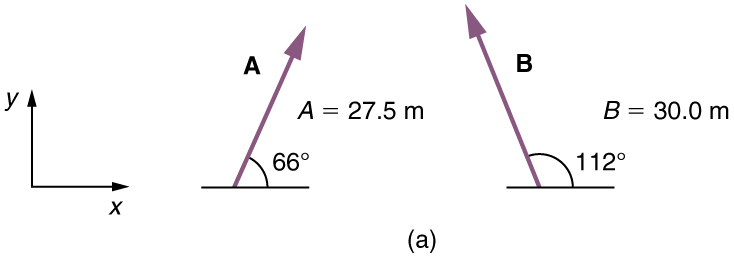Figure 14.

#### Strategy

We can represent the first leg of the trip with a vector A, and the second leg of the trip with a vector B. The dock is located at a location A + B. If the woman mistakenly travels in the opposite direction for the second leg of the journey, she will travel a distance B (30.0 m) in the direction 180º–112º=68º south of east. We represent this as –B, as shown below. The vector –B has the same magnitude as B but is in the opposite direction. Thus, she will end up at a location A + (–B), or A – B.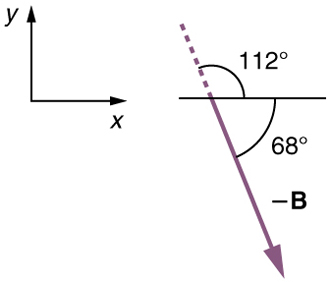Figure 15

We will perform vector addition to compare the location of the dock, A + B, with the location at which the woman mistakenly arrives, A + (-B).

#### Solution

(1) To determine the location at which the woman arrives by accident, draw vectors A and –B.

(2) Place the vectors head to tail.

(3) Draw the resultant vector R.

(4) Use a ruler and protractor to measure the magnitude and direction of R.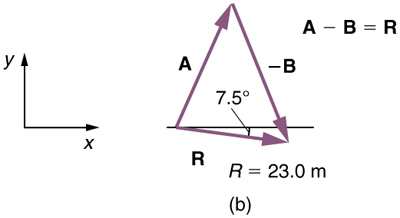Figure 16

In this case, R = 23 . 0 m and θ = 7 . 5º south of east.

(5) To determine the location of the dock, we repeat this method to add vectors A and B. We obtain the resultant vector R’: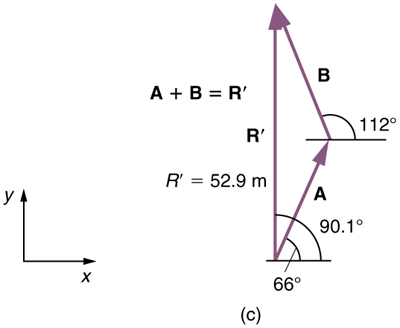Figure 17

In this case R = 52.9 m and θ = 90.1º north of east.

We can see that the woman will end up a significant distance from the dock if she travels in the opposite direction for the second leg of the trip.

#### Discussion

Because subtraction of a vector is the same as addition of a vector with the opposite direction, the graphical method of subtracting vectors works the same as for addition.

## Multiplication of Vectors and Scalars

If we decided to walk three times as far on the first leg of the trip considered in the preceding example, then we would walk 3 × 27.5 m, or 82.5 m, in a direction 66.0º north of east. This is an example of multiplying a vector by a positive scalar. Notice that the magnitude changes, but the direction stays the same.

If the scalar is negative, then multiplying a vector by it changes the vector’s magnitude and gives the new vector the opposite direction. For example, if you multiply by –2, the magnitude doubles but the direction changes. We can summarize these rules in the following way: When vector A is multiplied by a scalar c,

• the magnitude of the vector becomes the absolute value of cA,
• if c is positive, the direction of the vector does not change,
• if c is negative, the direction is reversed.

In our case, = 3 and = 27.5 m. Vectors are multiplied by scalars in many situations. Note that division is the inverse of multiplication. For example, dividing by 2 is the same as multiplying by the value (1/2). The rules for multiplication of vectors by scalars are the same for division; simply treat the divisor as a scalar between 0 and 1.

## Resolving a Vector into Components

In the examples above, we have been adding vectors to determine the resultant vector. In many cases, however, we will need to do the opposite. We will need to take a single vector and find what other vectors added together produce it. In most cases, this involves determining the perpendicular components of a single vector, for example the x and y-components, or the north-south and east-west components.

For example, we may know that the total displacement of a person walking in a city is 10.3 blocks in a direction 29.0º north of east and want to find out how many blocks east and north had to be walked. This method is called finding the components (or parts) of the displacement in the east and north directions, and it is the inverse of the process followed to find the total displacement. It is one example of finding the components of a vector. There are many applications in physics where this is a useful thing to do. We will see this soon in Projectile Motion, and much more when we cover forces in Dynamics: Newton’s Laws of Motion. Most of these involve finding components along perpendicular axes (such as north and east), so that right triangles are involved. The analytical techniques presented in Vector Addition and Subtraction: Analytical Methods are ideal for finding vector components.

## PhET Explorations: Maze Game

Learn about position, velocity, and acceleration in the “Arena of Pain”. Use the green arrow to move the ball. Add more walls to the arena to make the game more difficult. Try to make a goal as fast as you can.

## Summary

• The graphical method of adding vectors A and B involves drawing vectors on a graph and adding them using the head-to-tail method. The resultant vector R is defined such that A + B = R. The magnitude and direction of R are then determined with a ruler and protractor, respectively.
• The graphical method of subtracting vector B from A involves adding the opposite of vector B, which is defined as -B. In this case, A – B = A + (-B) = R. Then, the head-to-tail method of addition is followed in the usual way to obtain the resultant vector R.
• Addition of vectors is commutative such that A + B = B + A.
• The head-to-tail method of adding vectors involves drawing the first vector on a graph and then placing the tail of each subsequent vector at the head of the previous vector. The resultant vector is then drawn from the tail of the first vector to the head of the final vector.
• If a vector A is multiplied by a scalar quantity c, the magnitude of the product is given by cA. If c is positive, the direction of the product points in the same direction as A; if c is negative, the direction of the product points in the opposite direction as A.

### Conceptual Questions

1. Which of the following is a vector: a person’s height, the altitude on Mt. Everest, the age of the Earth, the boiling point of water, the cost of this book, the Earth’s population, the acceleration of gravity?

2. Give a specific example of a vector, stating its magnitude, units, and direction.

3. What do vectors and scalars have in common? How do they differ?

4. Two campers in a national park hike from their cabin to the same spot on a lake, each taking a different path, as illustrated below. The total distance traveled along Path 1 is 7.5 km, and that along Path 2 is 8.2 km. What is the final displacement of each camper?

5. If an airplane pilot is told to fly 123 km in a straight line to get from San Francisco to Sacramento, explain why he could end up anywhere on the circle shown in Figure 19. What other information would he need to get to Sacramento?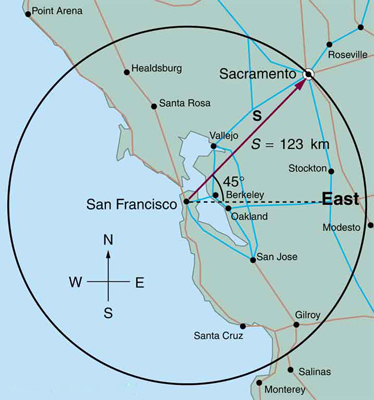Figure 19.

6. Suppose you take two steps A and B (that is, two nonzero displacements). Under what circumstances can you end up at your starting point? More generally, under what circumstances can two nonzero vectors add to give zero? Is the maximum distance you can end up from the starting point A + B the sum of the lengths of the two steps?

7. Explain why it is not possible to add a scalar to a vector.

8. If you take two steps of different sizes, can you end up at your starting point? More generally, can two vectors with different magnitudes ever add to zero? Can three or more?

### Problems & Exercises

Use graphical methods to solve these problems. You may assume data taken from graphs is accurate to three digits.

1. Find the following for path A in Figure 20: (a) the total distance traveled, and (b) the magnitude and direction of the displacement from start to finish.

2. Find the following for path B in Figure 20: (a) the total distance traveled, and (b) the magnitude and direction of the displacement from start to finish.

3. Find the north and east components of the displacement for the hikers shown in Figure 20.

4. Suppose you walk 18.0 m straight west and then 25.0 m straight north. How far are you from your starting point, and what is the compass direction of a line connecting your starting point to your final position? (If you represent the two legs of the walk as vector displacements A and B, as in Figure 21, then this problem asks you to find their sum R = A + B.

5. Suppose you first walk 12.0 m in a direction 20 west of north and then 20.0 m in a direction 40.0º south of west. How far are you from your starting point, and what is the compass direction of a line connecting your starting point to your final position? (If you represent the two legs of the walk as vector displacements A and B, as in Figure 22, then this problem finds their sum R = A + B.)

6. Repeat the problem above, but reverse the order of the two legs of the walk; show that you get the same final result. That is, you first walk leg B, which is 20.0 m in a direction exactly 40º south of west, and then leg A, which is 12.0 m in a direction exactly 12.0 west of north. (This problem shows that A + B = B + A.)

7. (a) Repeat the problem two problems prior, but for the second leg you walk 20.0 m in a direction 40º north of east (which is equivalent to subtracting B from A —that is, to finding R’ = A – B). (b) Repeat the problem two problems prior, but now you first walk 20.0 m in a direction 40º south of west and then 12.0 m in a direction 20º east of south (which is equivalent to subtracting A from B—that is, to finding R” = B – A = R’ Show that this is the case.

8. Show that the order of addition of three vectors does not affect their sum. Show this property by choosing any three vectors A, B, and C, and $\mathbf{C}$ , all having different lengths and directions. Find the sum A + B + C then find their sum when added in a different order and show the result is the same. (There are five other orders in which A, B, and C, can be added; choose only one.)

9. Show that the sum of the vectors discussed in Example 2 gives the result shown in Figure 17.

10. Find the magnitudes of velocities VA and VB in Figure 23.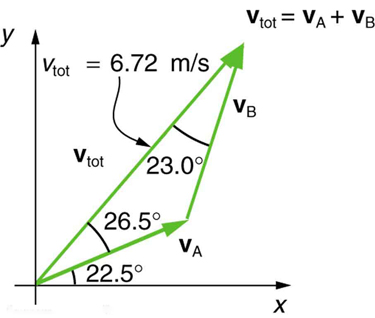Figure 23. The two velocities VA and VB add to give a total Vtot.

11. Find the components of vtot along the x– and y-axes in Figure 23.

12. Find the components of vtot along a set of perpendicular axes rotated 30º counterclockwise relative to those in Figure 23.

## Glossary

component (of a 2-d vector):
a piece of a vector that points in either the vertical or the horizontal direction; every 2-d vector can be expressed as a sum of two vertical and horizontal vector components
commutative:
refers to the interchangeability of order in a function; vector addition is commutative because the order in which vectors are added together does not affect the final sum
direction (of a vector):
the orientation of a vector in space
the end point of a vector; the location of the tip of the vector’s arrowhead; also referred to as the “tip”
a method of adding vectors in which the tail of each vector is placed at the head of the previous vector
magnitude (of a vector):
the length or size of a vector; magnitude is a scalar quantity
resultant:
the sum of two or more vectors
resultant vector:
the vector sum of two or more vectors
scalar:
a quantity with magnitude but no direction
tail:
the start point of a vector; opposite to the head or tip of the arrow

### Selected Solutions to Problems & Exercises

1. (a) 480 m (b) 379 m, 18.4º east of north

3. north component 3.21 km, east component 3.83 km

5. 19.5 m, 4.65º south of west

7. (a) 26.6 m, 65.1º north of east (b) 26.6 m, 65.1º south of west

9. 52.9 m, 90. with respect to the x-axis.

11. x-component 4.41 m/s, y-component 5.07 m/s